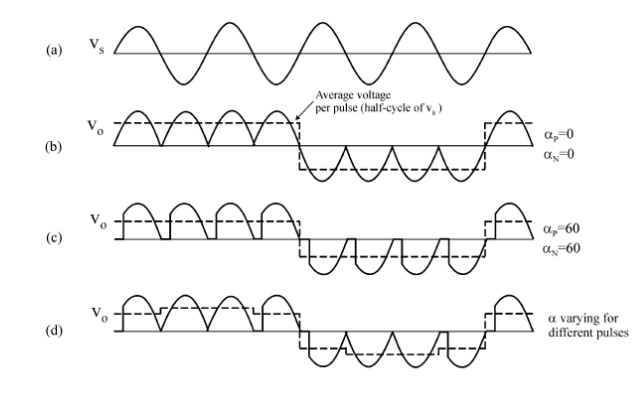Home | | Power Electronics | Single phase cycloconverter

# Single phase cycloconverter

This converter consists of back-to-back connection of two full-wave rectifier circuits. The input voltage, vs is an ac voltage at a frequency,

Single phase cycloconverter

This converter consists of back-to-back connection of two full-wave rectifier circuits. The input voltage, vs is an ac voltage at a frequency, For easy understanding assume that all the thyristors are fired firing angle, i.e. thyristors act like diodes. Note that the firing angles are named as  P for the positive converter and for the negative converter. Consider the operation of the cycloconverter to get one-fourth of the input frequency at the output. For the first two cycles of vs, the positive converter operates supplying current to the load. It rectifies the input voltage; therefore, the load sees 4 positive half cycles. In the next two cycles, the negative converter operates supplying current to the load in the reverse direction. The current waveforms are not shown in the figures because the resistive load.current will have the same waveform as the voltage but only scaled by the resistance. Note that when one of the converters operates the other one is disabled, so that there is no current circulating between the two rectifiersThe frequency of the output voltage, vo in Fig. 3b is 4 times less than that of vs, the input voltage, i.e. fo/fi=1/4. Thus, this is a step-down cycloconverter. On the other hand, cycloconverters that have fo/fi>1 frequency relation are called step-up cycloconverters. Note that step-down cycloconverters are more widely used than the step-up ones.

The frequency of vo can be changed by varying the number of cycles the positive and the negative converters work. It can only change as integer multiples of fi in 1f-1f cycloconverters.

With the above operation, the 1f-1f cycloconverter can only supply a certain voltage at a certain firing angle a. The dc output of each rectifier is:Thus varying a, the fundamental output voltage can be controlled.

Constant a operation gives a crude output waveform with rich harmonic content. The dotted lines in Fig. 3b and c show a square wave. If the square wave can be modified to look more like a sine wave, the harmonics would be reduced. For this reason a is modulated as shown in Fig. 3d. Now, the six-stepped dotted line is more like a sinewave with fewer harmonics. The more pulses there are with different a's, the less are the harmonics.

Study Material, Lecturing Notes, Assignment, Reference, Wiki description explanation, brief detail
Power Electronics : AC to AC Converters : Single phase cycloconverter |